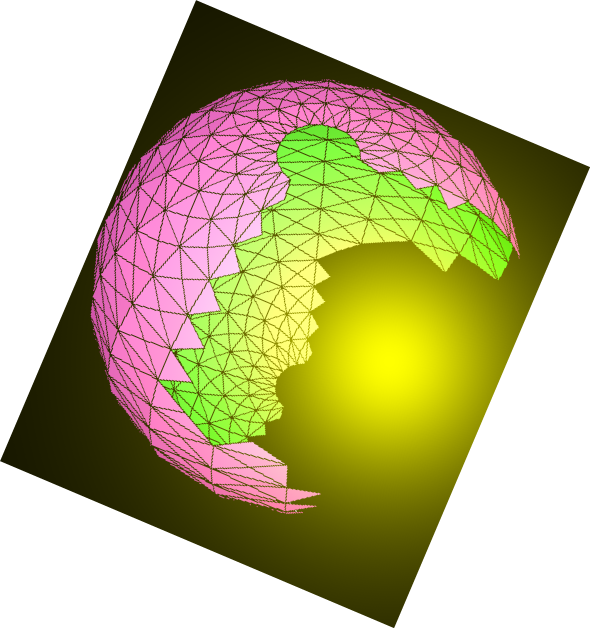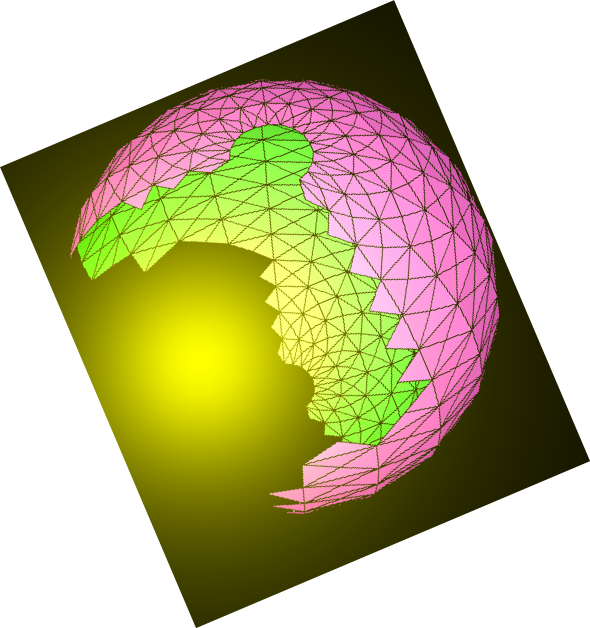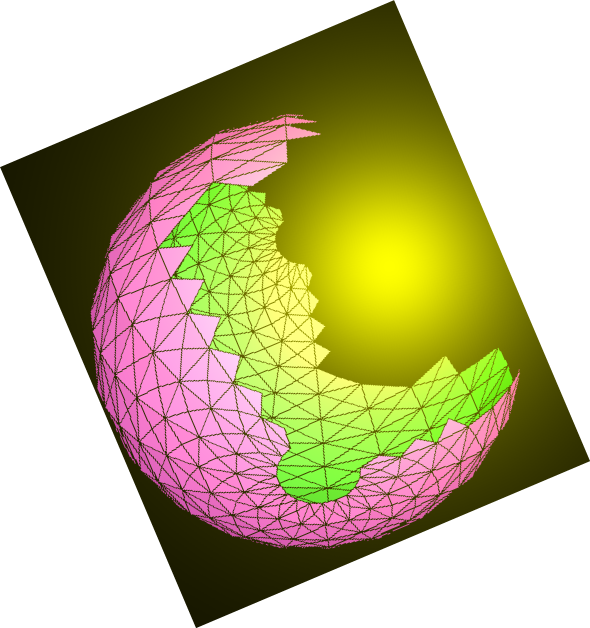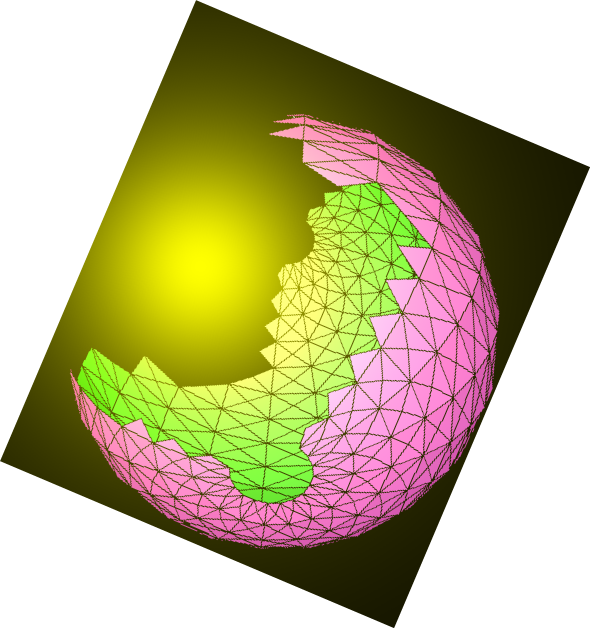# N.S.B. Cosmic Center### N.S.B. Files by Nazir S. Baaklini

Back to Books

The followings present a series of concise review articles that encompass most of fundamental theoretical physics at the advanced undergraduate, graduate, and higher levels. These articles bring out the basic concepts and emphasize the associated mathematical foundations.

These documents, taking the form of pdf files, may be downloaded from another web location that belongs to the author. You can obtain the needed links, and additional information, from the author at nazirbaaklini@yahoo.com.

#### NSBF-Alg-011 Matrix Algebra by N.S. Baaklini

We review the formalism of non-commutative matrix operators, introducing their associated manipulative rules of addition, multiplication, and taking inverses, the construction of matrix functions, and the processes of conjugation. We also treat the subjects of transformations, invariants, eigenvalues, variations of matrix expressions, as well as the composition and decomposition of matrices.

#### NSBF-Alg-012 Tensors and Coordinate Transformations by N.S. Baaklini

We review the formalism of tensors and their associated invariant operations, together with the concept of irreducible tensors and their description via Young tableaux. We treat the question of decomposing tensor products into irreducible parts. We present the theory of coordinate transformations and the construction of invariant densities using the concepts of metrics, exterior forms, and discuss infinitesimal transformations.

#### NSBF-Alg-013 Spinors and Dirac Algebra by N.S. Baaklini

We review the theory of spinors in a space of any number of dimensions. We discuss their description via Clifford and Dirac algebras, the irreducible decomposition of their products into irreducible parts, and their transposition properties.

#### NSBF-Alg-014 Lie Algebras by N.S. Baaklini

We review the formalism of transformation groups with continuous parameters and their underlying Lie-algebraic structure. We study the algebra of rotations in a space with three dimensions (or the angular momentum algebra) as a notable example of simple Lie algebras. We then introduce the classification theory of simple Lie algebras as well as their representation theory. Expressions for infinitesimal transformations and their relation to Lie algebraic structures are given.

#### NSBF-Alg-015 Orthogonal Algebras by N.S. Baaklini

We review the theory of orthogonal Lie algebras and present their tensorial and spinorial representations.

#### NSBF-Alg-016 Unitary Algebras by N.S. Baaklini

We review the formalism of unitary Lie algebras and present their tensorial representations. We discuss the isomorphic relations between some unitary algebras and orthogonal counterparts, as well as the unitary decomposition of orthogonal algebras.

#### NSBF-Alg-017 Symplectic Algebras by N.S. Baaklini

We review the formalism of symplectic algebras and their tensorial representations. We present the decomposition of the latter with regard to unitary subalgebras, and discuss the relation between symplectic and orthogonal algebras.

#### NSBF-Alg-018 Exceptional Algebra G2 by N.S. Baaklini

We present the exceptional Lie algebra G2 and its basic representations in terms of tensorial representations of SU3, its maximal subalgebra. Relations to other algebras are noted.

#### NSBF-Alg-019 Exceptional Algebra F4 by N.S. Baaklini

We present the exceptional Lie algebra F4, and its relation to other algebras.

#### NSBF-Alg-020 Exceptional Algebra E6 by N.S. Baaklini

We present the exceptional Lie algebra E6, its basic representations, and relations to other algebras.

#### NSBF-Alg-021 Exceptional Algebra E7 by N.S. Baaklini

We present the exceptional Lie algebra E7, its basic representations, and rela tions to other algebras.

#### NSBF-Alg-022 Exceptional Algebra E8 by N.S. Baaklini

We present the largest exceptional Lie algebra E8, its basic representations, and relations to other algebras. We express the algebra in terms of tensors pertaining to SU9, its maximal subalgebra, and also in terms of spinors pertaining to SO16, another maximal subalgebra.

#### NSBF-Alg-023 Superalgebras by N.S. Baaklini

We review the extension of the theory of Lie algebras to a counterpart containing both fermionic and bosonic generators. We examine the underlying system of commutators and anticommutators, and associated transformations with fermionic and bosonic parameters. We describe the possible simple superalgebras in their unitary, orthosymplectic, and exceptional classes.

#### NSBF-CM-001 Elementary Mechanics by N.S. Baaklini

The subject of mechanics is introduced at an elementary level. We treat the description of particle motion via the concepts of coordinates, velocity, and acceleration, and introduce the concepts of momentum and force. Newton's laws of motion and that of gravitation are introduced. We discuss the cases of motion at constant acceleration, circular motion, and a Keplerian law for orbital motion. Several elementary examples of motion including fall under gravitation, oscillator motion, and frictional effects are considered.

#### NSBF-CM-003 Spherical Coordinates and 3D Motion by N.S. Baaklini

We review the motion of a particle in 3 dimensions using vectorial formalism. Topics include the motion of a particle under a central force, and the associated orbital equation.

#### NSBF-CM-005 Introduction to Fluid Mechanics by N.S. Baaklini

We present an elementary introduction to the equations of fl uid mechanics comprising Euler's equations, the equations of continuity, conservation laws, and Bernoulli's equation.

#### NSBF-CM-011 Lagrangian and Hamiltonian Principles by N.S. Baaklini

We introduce the Lagrangian formalism and the associated minimal action principle for the description of motion, derive the Euler-Lagrange equations, and discuss their relation to the Newtonian equations. The transition to the Hamiltonian formalism and the associated phase-space framework is then described with the derivation of the Hamiltonian equations. We discuss the canonical transformations and the derivation of the Hamilton-Jacobi equation, together with Poisson brackets, and infinitesimal transformations, notably space-time translations and angular momenta. We introduce Dirac's dynamics for constrained systems, and describe the generalization to systems with both fermionic and bosonic coordinates.

#### NSBF-Data-001 Physical Constants by N.S. Baaklini

This gives a collection of fundamental physical constants as well as derived counterparts that are important to any physical theorist.

#### NSBF-EM-011 Electrostatics by N.S. Baaklini

This reviews the basic equations of electrostatics starting with electrostatic force, then proceeding to the concepts of electric field and electric potential. The fundamental differential equations for the electric field and potential are given, and expressions for the electrostatic energy associated with a system of charges, and the energy density associated with an electric field, are derived.

#### NSBF-EM-012 Magnetostatics by N.S.Baaklini

Starting with the concepts of electric currents and current densities we give expressions for the magnetic fields and magnetic forces that are produced by and that act on them. We then give expressions for the vector potential associated with such the magnetic fields, and derive the differential equations that are associated with the magnetic field and potential. Expressions for the magnetostatic energy associated with configurations of current elements and current densities, and for the energy density corresponding to a magnetic field, are derived.

#### NSBF-EM-013 Electrodynamics by N.S. Baaklini

We present Faraday's empirical law of induction and derive the corresponding differential equation for the underlying electric and magnetic fields. We then proceed to present the full set of Maxwell's electromagnetic field equations. The latter equations are used to derive the corresponding electromagnetic wave equations and their solutions, identifying the speed of these waves given in terms of electric and magnetic constants with the speed of light. We then derive the continuity equation that relates electromagnetic energy density to the current density associated with the ow of electromagnetic energy. The dynamics of a charged particle that is coupled to electric and magnetic potentials are derived from Lagrangian principles giving an expression for the Lorentz force, and setting up the associated Hamiltonian dynamics.

#### NSBF-EP-011 Introduction to Subnuclear Phenomenology by N.S. Baaklini

We describe the advent of subnuclear physics and the introduction of nuclear isospin to be associated with nucleons and pions. The proliferation of hadronic particles is then discussed. We also describe the advent of the neutrino and the introduction of the Fermi coupling.

#### NSBF-EP-012 Subnuclear Particles and Interactions by N.S. Baaklini

We describe the classification of hadronic particles in terms of an internal SU3 symmetry, discuss associated selection rules for subnuclear processes, and what's beyond SU3. We move to describe the advent of quarks in the description of hadronic structure, and the correspondence between quark generations and leptonic counterparts. The universal (V-A) form of weak interaction is presented, and the concept of an intermediate vector boson is introduced.

#### NSBF-EP-013 Electronuclear Gauge Theory by N.S. Baaklini

We review the construction of a gauge theory of strong interactions based on the SU3 quark color degree of freedom (quantum chromodynamics), as well as the construction of an SU2xU1 gauge theory of elecromagnetic and weak interactions (electroweak). We discuss the underlying mechanism of symmetry breaking and the effective low-energy phenomenology.

#### NSBF-EP-014 Quark-Lepton Unification in SU5 and Alternatives by N.S. Baaklini

We review the ideas of quark-lepton unification, notably within the framework of a simple Lie algebra, such as SU5, and associated gauge theoretic framework. We describe the implications of such a scheme together with symmetry breaking, low-energy phenomenology, and proton decay. Alternative schemes bases on SUN>5, exceptional algebras, and notably supersymmetric unification under E8 are also described.

#### NSBF-FT-011 Algebraic Gauge Principles by N.S. Baaklini

We present the general scheme of constructing locally invariant field systems associated with simple Lie algebras. We discuss the general scheme of generating symmetry-breaking vacuum solutions, and provide some examples regarding orthogonal, unitary, symplectic, and exceptional gauge systems.

#### NSBF-FT-012 Supersymmetric Gauge Field Theory by N.S. Baaklini

We review the construction of supersymmetric gauge theory using the formalism of superfields, and discuss the problem of symmetry breaking.

#### NSBF-FT-013 Extended Spacetime Symmetries and Supersymmetries by N.S. Baaklini

We review the extension of the Poincare superalgebra by using several fermionic generators, either employing some internal symmetry index (2, 4, and 8-fold), or through increasing the number of spacetime dimensions (6, 10 and 11-D). The constructions of corresponding supermultiplets and associated invariant Lagrangians are given. We also give the extension of the Poincare algebra to a conformal algebra and a conformal superalgebra.

#### NSBF-FT-014 Einstein's Gravidynamic Field Theory by N.S. Baaklini

We treat the construction of field theory actions that are manifestly Einstein invariant. We introduce the concepts of covariant densities, the Einstein connection, as well as the curvature tensor and its contractions. We present the Einstein action for the gravitational field, its couplings to scalar and vector fields, as well as the associated variational principles.

#### NSBF-FT-015 Einstein-Dirac Gravidynamics by N.S. Baaklini

We present the construction of Einstein dynamics for the Dirac field. The associated vierbein formulation, and its relation to the metric theory, will be discussed. The theory is formulated in a locally Lorentz invariant framework. The transcending Poincare gauge structure is also remarked upon. We finally display the elegant frame-independent structure of the vierbein theory and its topological invariance.

#### NSBF-FT-016 Supergravidynamics by N.S. Baaklini

The theory of supergravity (supergravidynamics) extends gravidynamic field theories into locally supersymmetric counterparts. In its simplest version, the theory associates a spinor-vector fermionic field (spin-3/2) as a superpartner, termed gravitino, to the symmetric tensor (spin-2) field describing the graviton. Extended versions introduce several gravitinos, as well as other lower-spin particles, all as partners to the graviton in a unified supermultiplet. We shall describe the simplest supergravidynamic model. We shall display its topological structure, discuss some extensions, present the rather incomplete description using auxilliary fields, and in superspace.

#### NSBF-FT-017 Gravidynamic Theory in Higher Dimensions by N.S. Baaklini

We present the extension of Einstein's gravidynamic field theory to higher-dimensional spacetimes, treating the Kaluza-Klein 5-dimensional formulation that aimed to encompass electromagnetism, and the higher extensions that deal with non-Abelian gauge fields. We also present the higher-dimensional Einstein-Dirac theory, as well as the 11-dimensional supergravity theory.

#### NSBF-QM-011 Principles of Quantum Mechanics by N.S. Baaklini

The basic principles of quantum mechanics are reviewed, together with the concept of matter waves, the associated (Schrodinger) wave equation and wave function, the probability density and corresponding current, and the expectation values. The wave equation for the hydrogen atom is set up and solved, giving the energy spectrum. The extension of the principles to matter fields is presented.

#### NSBF-QM-012 The Dirac Equation by N.S. Baaklini

We review Dirac's construction of the relativistic wave equation that implements electron spin, in terms of 4x4 matrices, and how the associated wave function describes the electron and the positron. We present the coupling of the equation to the electromagnetic (or photon) field, the resulting magnetic moment of the electron, and the associated probability density and current conservation.

#### NSBF-RT-011 Einstein Mechanics and Maxwell's Electrodynamics by N.S. Baaklini

We review the foundations of Einstein's relativistic mechanics in the framework of a Lagrangian principle and Minkowskian spacetime, then describe Maxwellian electrodynamics in the corresponding 4-dimensional notation.

#### NSBF-RT-014 The Lorentz-Poincare Algebra by N.S. Baaklini

We present the Lorentz-Poincare algebra and its tensorial and spinorial field representations.

#### NSBF-RT-015 Relativistic Fields and Action Principles by N.S. Baaklini

We present the fundamental fields of low spins 0, 1/2, 1, 3/2, 2 as representations of the Lorentz-Poincare algebra. We discuss actions principles and refl ective symmetries that are associated with these fields.

#### NSBF-RT-016 The Poincare Superalgebra and Superfields by N.S. Baaklini

We review the simplest superalgebraic extension of the Poincare superalgebra with the introduction of a fermionic Majorana spinor generator. The representations of such an extension via supermultiplets and superfields that describe both fermionic fields and bosonic fields are described, giving the associated formalism in detail.

#### NSBF-TS-011 Principles of Thermodynamics and Statistical Mechanics by N.S. Baaklini

The basic principles of thermodynamics and the associated empirical laws are reviewed, with the underlying concepts of temperature, pressure, heat, entropy, and thermodynamic energy functions. Also reviewed are the basic principles of statistical thermodynamics at the classical and at the quantum levels. The latter principles are applied to the system of a harmonic oscillator.

#### NSBF-TS-012 Ideal Gases by N.S. Baaklini

We review the statistical thermodynamics of an ideal gas at the classical and the quantum levels. We treat the respective cases of a Bose and a Fermi gase, as well as a relativistic gas. We disuss Bose-Einstein condensation, and the relation between entropy and information.

Many more such review articles will appear in due course,